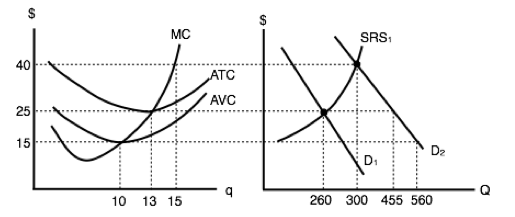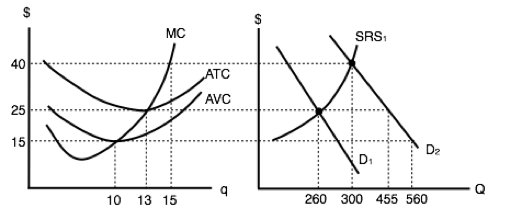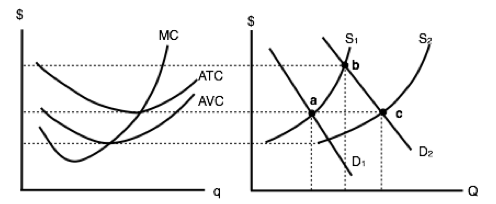Topic 7: Producer Theory

# 7.3 Producer Theory in the Long Run

### Learning Objectives

By the end of this section, you will be able to:

• Understand why positive and negative profits entice entry and exit.
• Differentiate between accounting and economic profits.
• Explain what happens in the long-run after shocks to supply and demand.

So far we have looked at The Clip Joint in the short-run, while eluding to some of the actions The Clip Joint may take in the LR including:

• If the price is below The Clip Joint’s break-even price, it will continue to operate in the short-run but exit in the long run.
• If the price is below The Clip Joint’s shut-down price, it will shut down in the short-run.

In our example in Topic 7.2, when price was \$7.50, we showed The Clip Joint making positive profits in the short run. To begin our discussion of long-run, we must first remember that The Clip Joint is a price-taker who is receiving price cues from the competitive market. This means that the price that The Clip Joint faces is equal to the equilibrium price in the aggregate market. In Figure 7.3a, the competitive market is shown with an equilibrium price of \$7.5, and an equilibrium quantity of 5,500 haircuts. Assuming every firm is identical to The Clip Joint, we can determine how many firms are in the market by the equation Q/q, dividing the 5,500 haircuts produced in the market, by the 110 haircuts each firm produces at the market price. This shows us that in this market, there are 5,500/110 = 50 firms.

In this situation, cutting hair is an attractive industry. Each firm is able to pay all variable costs (which include opportunity costs) and fixed costs and make \$210 in profit after economic expenses! In the long run, this is unsustainable – other businesses will see the profitable market and decide to join the industry. This activity will cause supply to increase.

In Figure 7.3b, our supply has increased from S1 to S2, causing price to fall from \$7.5 to \$6.5 and creating a new equilibrium quantity of 6,600. Consider what this has done for The Clip Joint’s profits. Now, with the market change, The Clip Joint is making only \$52.50 in profits – a significant decrease from before. Notice that the amount of firms has increased from 50 to 6,600/105 = 63 firms.

Although the number of firms has increased and the equilibrium price has fallen, there is still incentive in the market for firms to enter. Since profits are positive, supply will continue to increase.

Supply continues to increase until Price = \$5.8, which is the break-even price for the market. At this point, The Clip Joint is making no profits and there is no incentive for other firms to enter.

In this analysis, we have shown that when a firm is making positive profits in the short-run, in the long run, this will cause more firms to enter, decreasing price until it is at the break-even point once more. If price is below break-even, the reverse will occur as firms leave the market to seek opportunities elsewhere. The important take away is:

While firms can make positive or negative profits in the short-run, in the long run, firms will always break-even.

## No Economic Profits?

How can it be that in the long-run equilibrium firms make no profits? Are we suggesting that no firms can make any money in the long-run? These questions stem from the difference between Accounting Profits and Economic Profits.

Accounting profits are what most of us think of when we hear “profits.” This is the bottom line of the income statement equal to our firm’s revenues minus expenses. Economic profit on the other hand includes opportunity costs. This means that our accounting profits could be giving us 6% returns, and our economic profits could be zero if we could be making that same 6% elsewhere.

In conclusion, firms can certainly make accounting profits in the long-run, but their economic profits will always return to 0.

## Comparative Statics

You now know all the basic tools for producer theory, with the understanding of how a single firm behaves in a market, and how a market responds when profits are not 0. Let’s conclude by applying these concepts to the comparative statics exercises we learned in Topic 3.

In the market for haircuts, consider what would happen if a new peer-reviewed study was released saying that people with hair shorter than 6 inches are 60% more likely to catch the common cold in a given year. Considering what we learned in Topic 3, we know that this would decrease the demand for haircuts, as many consumers would prefer long hair over getting sick.

As shown in Figure 7.3d, this causes a decrease in demand, which causes price to fall from \$5.80 to \$4.00 and quantity to drop from 7,500 to 6,000 haircuts moving from E1 to E2. At this price, The Clip Joint will reduce services from 100 to 80 haircuts per day. In addition, with ATC as high as \$6.20, The Clip Joint is losing \$168 per day. Since The Clip Joint is able to cover its variable costs, it will remain in the market in the short-term, but once it’s lease runs out, The Clip Joint will close down.

Consider the number of firms in the industry. In our previous example, we saw that changes in supply caused the number of firms to fluctuate. Before the demand shock, we had 75 Firms (7,500/100) and after, we still have 75 (6,000/80). Since each firm has reduced its production, all are able to stay in the market in the short-term.

In the long run, The Clip Joint, along with many other barbershops, leave the market. This causes supply to decrease, reducing the aggregate demand and raising the price. This creates a new equilibrium at E3, with EQ of 4,000 and EP of \$5.80.

Notice the EP has returned to the level it started at – this is no coincidence.

The competitive market always returns zero profits for its individual firms in the long-run, with entry and exit correcting any short-term deviations.

Since profits were negative, exit caused price to increases again until they were 0. When profits are positive, entry causes price to fall until they are 0. Just as we saw market corrections for shortages and surpluses, the competitive market will always return to an equilibrium where no firm is making positive economic profits.

## Glossary

Accounting Profits
total revenues minus explicit costs, including depreciation
Economic Profits
total revenues minus total costs (explicit plus implicit costs)
Entry
when firms enter a market enticed by positive profits, causes prices to fall
Exit
when firms leave a market due to losses, causes prices to rise

### Exercises 7.3

1. Suppose that a perfectly competitive firm is currently producing 10 units of output. You also have the following additional information:

P = \$6; MC; = \$6; AVC = \$3; ATC = \$8.

Given this:

a) This firm should continue to produce 10 units in the short run, but should exit the industry in the long run, unless price rises.
b) This firm should shut down in the short run, and exit the industry in the long run, unless price rises.
c) This firm should increase its price in order to maximize profits.
d) This firm should increase its output in order to maximize profits.

2. Suppose that a perfectly competitive industry is initially in long run equilibrium.

The government then introduces a \$1,000 annual fee that each firm in the industry must pay, no matter how much output it produces. Which of the following statements is TRUE?

a) Price will increase in the short run, but return to its initial level in the long run.
b) Price will not change in the short run, but will increase in the long run.
c) Price will increase in both the short and the long run, but the increase will be greater in the short run than in the long run.
d) Price will increase in both the short and the long run, but the increase will be greater in the long run than in the short run.

3. Suppose you are given the following information about a competitive firm:

P = \$10; ATC = \$4; MC = \$10; q = 20.

Given this:

a) The firm should continue to produce 20 units of output; this is profit maximizing.
b) In order to maximize profits, the firm should produce more than 20 units of output.
c) In order to maximize profits, the firm should produce fewer than 20 units of output.
d) The firm should shut down in the short run and exit in the long run.

4. Which of the following statements about competitive firms is FALSE?

a) A competitive firm maximizes profit by producing the level of output at which price equal marginal cost.
b) A competitive firm maximizes profit by producing the level of output at which marginal revenue equals marginal cost.
c) For a competitive firm, marginal revenue is less than price.
d) For a competitive firm, economic profits equal zero in the long run.

5. Suppose you are given the following information about a competitive firm:

P = \$10
ATC = \$4
MC = \$6
q = 20.

Which of the following statements is true?

a) The firm should continue to produce 20 units of output; this is profit maximizing.
b) In order to maximize profits, the firm should produce more than 20 units of output.
c) In order to maximize profits, the firm should produce fewer than 20 units of output.
d) The firm should shut down in the short run and exit in the long run.

6. Suppose that a perfectly competitive firm is currently producing 25 units of output. You also have the following additional information:

P = \$12.
The firms is operating at the minimum point of AVC
AVC = \$4.
ATC = \$10.
The minimum point of ATC is where ATC = \$8.

If the firm wishes to maximize profits, which of the following statements is TRUE?

a) This firm should continue to produce 25 units in the short run, but exit in the long run.
b) The firm should increase output in the short run, but exit in the long run.
c) The firm should increase output in the short run, and will remain in the industry in the long run.
d) None of the above.

7. Which of the following statements about a competitive firm producing positive output is TRUE?

I. Price equals marginal cost in both the short run and the long run.
II. Price can be less than average total cost in the short run, but not in the long run.
III. If price is less than average total cost (so that the firm makes negative economic profits) it will shut down in the short run and exit in the long run.

a) I only.
b) I and II only.
c) I and III.
d) I, II, and III.

The following THREE questions refer to the diagram below, which illustrates a competitive firm’s cost curves and the supply and demand diagram for the industry. Assume that all firms in the industry have identical cost curves.8. If demand is given by D1, then each firm in the industry will produce _____ units of output.

a) 0.
b) 10.
c) 13.
d) 15.

9. If demand is given by D1, then how many firms operate in this industry?

a) There is insufficient information to determine the number of firms in the industry.
b) 10.
c) 20.
d) 30.

10. If demand shifts from D1 to D2, then, in the short run:

a) Firms will make positive economic profits.
b) New firms will enter the market.
c) The price will remain unchanged at \$25.
d) Some firms will stop producing, since price is below average variable cost.

11. Suppose that the wages competitive firms must pay to their workers increase. Which of the following statements is TRUE?

a) The firms’ marginal costs will rise.
b) The market supply curve will shift left and price will rise.
c) In the long run firms will be making zero economic profits.
d) All of the above are true.

The following TWO question refers to the diagram below, which illustrates a competitive firm’s cost curves, and the supply and demand diagram for the industry. Assume that all firms in the industry have identical cost curves.12. If demand increases from D1 to D2, then, in the long run, how many firms will be in this industry?

a) 20.
b) 25.
c) 35.
d) 40.

13. If demand increases from D1 to D2, then, in the short run price will ______, and in the long run price will _____.

a) Rise to \$40; remain at \$40.
b) Rise to \$40; fall to \$25.
c) Rise to \$40; fall to \$15.
d) Rise to \$40; either rise or fall, depending on whether firms enter or exit.

14. Refer to the diagram below.Which of the following labeled points is a long run equilibrium?

I. Point a.
II. Point b.
III. Point c.

a) I only.
b) I and II only.
c) I and III only.
d) I, II, and III.